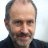# Problem with flags

J

#### Jompatan

##### New member
Hello, I am new to all this, I have the following problem:
I need that from a certain condition fulfilled (A) I can do an operation only once (in this case add 1 point to the counter), and then do another different operation (in this case add 10 points to the counter).
The problem is that I cannot modify a variable that is used in the IF condition.

def A=1;
def counter;
if A==1 {
counter= 1+counter;
A=0;
}else{
counter= 10+counter;
}
plot prueba= A;

Value never assigned to a at 6:1
Value never assigned to a at 6:1#### inthefutures

##### Active member
def counter = if A == 1 then 1 + counter else if a==0 then 10 + counter else 0;
plot prueba = counter;

not a lot of info to go on but try this

J

#### Jompatan

##### New member
Thanks for answering, but actually I need to do many more operations within the true and false condition.

def A = 1;

if A == 1 {
MANY OPERATIONS
A = 0;----------------------->>>> Here I want to change the value of A so that after doing the operations on the true side, it will never enter again.
} else {
MANY OPERATIONS
}#### inthefutures

##### Active member
left me confused so think you need to be more specific#### horserider

##### Well-known member
VIP
It's a secret.J

#### Jompatan

##### New member
Ok, I want to try a strategy with backtesting and as an example I am going to assume the following exercise (remember that it is an example):
I have a starting capital of \$ 1000 and I am going to trade long on all the bars (just to understand it). The first operation will be (1000 / shares purchased) * profit.
From the second my capital will no longer be 1000, and that's where my problem begins to know the new profits according to the new capital.

declare lower;
input investment = 1000;
def profit = close-open;
def A = 1 + A ;
def total;

if A == 1 {
total = round((investment/ open), 0) * profit;
} else {
total = round((total / open), 0) * profit;
}
def totals = total + total;

plot data = totals;

This example doesn't throw any error in the code, but it doesn't show results, so there is something wrong.Percent Range Flags Turning Points for thinkorSwim Indicators 1